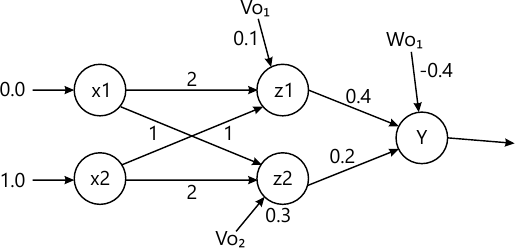MU Information Technology (Semester 8)
Soft Computing
December 2015
Total marks: --
Total time: --
INSTRUCTIONS
(1) Assume appropriate data and state your reasons
(2) Marks are given to the right of every question
(3) Draw neat diagrams wherever necessary

Solve any four
1 (a) Define soft computing? Distinguish between soft computing and hard computing.
5 M
1 (b) Determine (alpha) α-level sets and strong α-level sets for the following fuzzy set.
A = {(1, 0.2), (2, 0.5), (3, 0.8), (4, 1), (5, 0.7), (6, 0.3)};
5 M
1 (c) Prove that the first order derivative of a unipolar continuous activation function f' (net) = 0 (1-0)
5 M
1 (d) Draw the five layer architecture of ANFIS and explain each layer in brief.
5 M
1 (e) What are the differences between derivative free and derivative based optimization.
5 M
1 (f) Distinguish between Supervised and Un-supervised learning.
5 M

2 Design a fuzzy controller for a train approaching station. Inputs are speed and Distance and output is Break power. Use triangular membership function. Consider two descriptor for input and three descriptors for output. Derive a set of rules for control action and de-fuzzification. The design should be supported by figures wherever possible. Design a fuzzy controller for a train with high speed and small distance.
20 M

3 (a) Apply Back propagation Algorithm to find the final weights for the following net. Inputs x={0.0, 1.0}, Weights between Hidden and output Layers: w={0.4, 0.2}, Bias on the Output Node O is Wo=[-0, 4], Weights between Input and Hidden Layer: v={2, 1; 1, 2], Bias on Hidden Unit modes are Vo={0.1, 0.3}m desired output: d=1.0.10 M
3 (b) What is selft-organizing map? Draw and explain architecture of Kohonen Self Organization Feature Map KSOFM.
10 M

4 (a) What are the different types of encoding, selection, crossover, mutations of GA. Explain each type with suitable examples.
10 M
4 (b) Explain with suitable examples Linearly and Non-linearly separable pattern classification.
10 M

5 (a) Explain Learning Vector Quantization Algorithm?
10 M
5 (b) The formation of algal solutions in surface water is strongly dependent on pH of water, temperature and oxygen content. T is a set of water temperature from a lake given by T={50, 55, 60} and O is oxygen values in water given by O={1, 2, 6}. The fuzzy set of T is given by {0.7 / 50+0.8 / 55+0.9 / 60} and fuzzy set of O is given by {0.1 / 1+0.6/ 2+0.8 /6}
i) Find R=T×O for Given I=(0.5/ 50 +1/ 55+0.7/60}
ii) Find S=I o R using max- product composition
iii) Find S= I o R using max-min composition.
10 M

Write short notes on any two:
6 (a) Steepest Descent algorithm.
10 M
6 (b) Newton Method
10 M
6 (c) Fuzzy inference system.
10 M

More question papers from Soft Computing